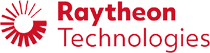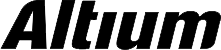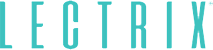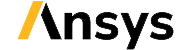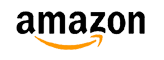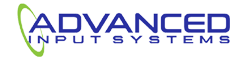﻿ How Your Digital Signal Bandwidth Affects Your PCB | NWES Blog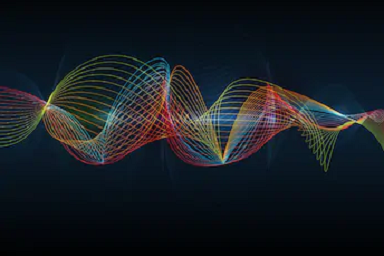By

The ideal digital waveform looks very simple, but real digital signals can have complex behavior in the time domain. This behavior in the time domain is reflected in the frequency domain, and one needs to define a digital signal bandwidth for use in circuit design. The bandwidth of a digital signal and the bandwidth of a circuit will determine whether a digital signal becomes distorted.

Understanding the digital signal bandwidth is also important beyond circuit design. In your PCB, dispersion in the dielectric substrate will influence how different frequency components in a signal behave as they propagate. This also causes signal distortion as a pulse is stretched and attenuated during propagation. Addressing both points requires calculating the digital signal bandwidth, which is not such a straightforward exercise. Here are some ways to understand digital signal bandwidth and how to use this in PCB design to ensure signal integrity.

## Calculating Digital Signal Bandwidth

To begin, the fundamental mathematical tool used for calculating the digital signal bandwidth is the Fourier transform operation. It’s important to understand that complex signal integrity simulation tools are not needed to calculate the bandwidth of a digital signal as long as you know its time-domain waveform. You can calculate the bandwidth of any signal in the time domain using a Fourier transform. Some time-domain waveforms are simple enough that you can calculate the expression for the Fourier spectrum by hand. In other cases, such as with multilevel time-domain signals with complex modulation, the Fourier transform needs to be calculated numerically.

The exact digital signal bandwidth you’ll see in the frequency domain depends on how you model your digital signals. In reality, the digitals signal bandwidth extends out to positive and negative infinity, and the bandwidth of a digital signal is not a specific number. As we’ll see, no matter how the time-domain waveform is defined, you’ll have to truncate the power spectrum of your signals at some point and take that limit as the edge of the digital signal bandwidth.

### Single Square Pulse

A two-level digital signal is essentially a square pulse with finite width in time. The Fourier transform of a square pulse is a complex sinc function, as shown in the graph below. Although the time-domain waveform is finite and ends at some specific time, the sinc function extends out to infinity.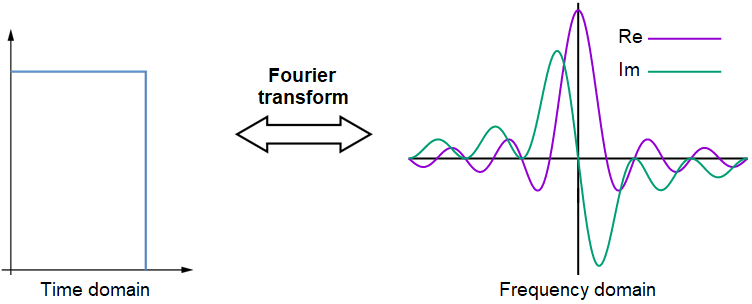A Fourier transform of a time-domain digital signal gives the digital signal bandwidth in the frequency domain. The frequency domain spectrum extends out to infinity. This graph shows the Fourier transform for a single square pulse, which is a sinc function.

The sinc function shown above is normally shown as a power spectrum or as an absolute value; the lobes in the power spectrum all lie above zero. The digital signal bandwidth in this case can be taken up to the frequency that includes ~75% of the total power in the signal. This particular frequency is called the 'knee frequency,' which will be discussed in more detail below.

### Stream of Square Pulses

For a stream of square pulses, the digital signal bandwidth is no longer a sinc function. Instead, you have a sum of sinc functions that are shifted in time and converge to a sum of sines and cosines. The standard formulation of Fourier series, which uses orthonormal sine and cosine functions, is used to represent a stream of square pulses with definite repetition frequency, edge rate, and amplitude. This gives the Fourier transform shown below. For a stream of repeating digital pulses with 50% duty cycle and infinite edge rate, the Fourier transform will be a set of specific frequencies with defined relative amplitudes.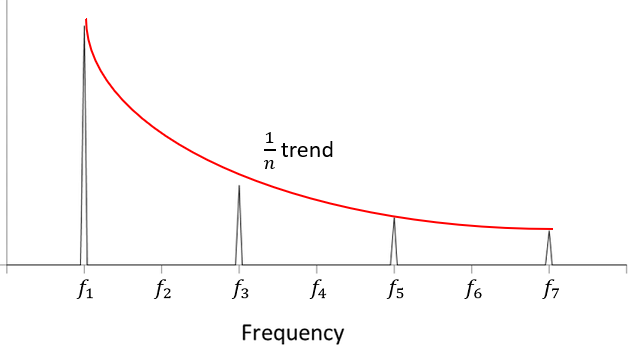Fourier transform of a stream of square pulses in the time domain.

Again, because the digital signal bandwidth extends out to infinity, we need to select some cutoff value and take this as the edge of the signal bandwidth. Most designers simply take the 5th harmonic, or 5x the repetition rate (i.e., the clock rate). This is fine for a signal with infinite edge rate as this harmonic will cover a decent portion of the power contained in the digital signal. However, real digital signals do not have infinite edge rate. As a result, the frequency covering up to ~75% of the power spectrum will depend on the edge rate, thus the edge rate is normally used to calculate some limiting bandwidth for a digital signal.

## Context For the Knee Frequency

One metric you will often see for use as a limit on bandwidth for a 2-level signal is the signal's knee frequency. This value was never meant to be used as a measure of digital signal bandwidth, and yet many designers continue to quote this as the value of bandwidth for a signal. This value for bandwidth is normally given by: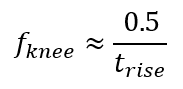If we had a factor 0.35 instead of the 0.5 factor, this would be equal to the -3 dB frequency for a capacitively terminated channel. All digital logic circuits have capacitive input impedance, and when they are sourced with a square wave input, the resulting response will appear as a charging/discharging capacitor. These two frequencies are derived assuming a critically damped rising/falling response as measured at the receiver input.

It is true that the rise time of a signal does influence its power spectrum, and thus some -3 dB bandwidth limit. The integral of the power spectrum from the repetition rate up to the knee frequency will contain ~75% of the signal power for a vanilla binary signal. Note that this is an arbitrary metric, but it is an easy way to set a limit on digital signal bandwidth that everyone can understand. However, this is only a relevant value to use in certain cases of high-speed digital channel design when the system is sourcing a standard square wave signal (e.g., SPI or I2C), and in many cases the channel will have plenty of bandwidth. Many examples can be found where this is not the case because modulation is being used to transfer digital data with an analog carrier; Ethernet is the most common example.

The knee frequency is also defined in terms of an oscilloscope's front-end, where it is the minimum amount of bandwidth an oscilloscope requires in order to resolve a digital signal without non-causal Gibbs artifacts (preshoot) assuming the oscilloscope's input filter stage is a 1st order filter. In reality, most digital oscilloscopes have at least a 4th order filter, so the above definition is not so useful for actual channel design. What matters is the minimum amount of bandwidth a channel needs to provide for a given data rate, rather than looking at this from the perspective of rise time. The reason is that rise times can differ in different systems, even though the resulting bitstream has the same data rate. As long as the channel does not excessively attenuate the signal's higher frequency components (i.e., provides some minimum bandwidth), then the receiver will be able to recover data in the bitstream.

## Nyquist Frequency From Data Rate

The standard metric to use to determine the amount of bandwidth required in a channel when the sourced signal is a modulated bitstream is the Nyquist frequency. Suppose a driving component is attempting to inject a bitstream with a specific data rate (D) into a channel. The minimum amount of bandwidth a channel requires is given by the formula below and depends on the number of signal levels (N) per unit interval (UI):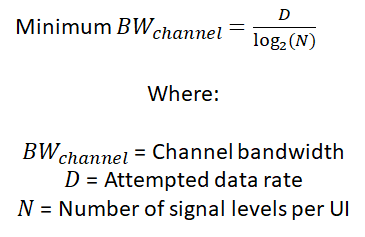In the above formula, you can determine the minimum required amount of bandwidth as long as you know the data rate you are attempting to work with and the modulation format. Currently, the two common modulation formats being used with the highest data rate digital bitstreams are PAM-4 and NRZ/RZ. If you want to transfer a bitstream with data rate D over a channel with NRZ modulation, then the minimum channel bandwidth would be D/4.

Next, the receiver used to capture a high-data-rate differential channel will define another frequency for that bitstream: the Nyquist frequency. The channel bandwidth should be at least equal to this frequency in order for the receiver to be able to resolve a bitstream. How do we determine whether a channel can support some minimum data rate? We have to look at the S-parameters for the channel. Generally, we have two metrics we want to use:

• Return loss (S11): For transmission lines, this measures the input impedance looking into the signal launch into the line. As long as the input impedance is closely matched to the target impedance within the required channel bandwidth, then the channel can support propagation into the channel at the required data rate.
• Insertion loss (S21): This will determine how much power is lost after the signal or bitstream propagates into the channel. Typically there will be some loss budget that is determined by the particular signaling standard or the receiver's sensitivity threshold.

The specific bandwidth limit and loss budget requirements are generally taken alongside crosstalk requirements, but overall these will be dictated by the particular signaling standard being investigated.

An example for a high-data-rate differential channel is shown below. This S11 graph tells us the degree to which the channel's input differential impedance matches some target impedance, and it is used to evaluate connector transitions, BGA footprint transitions, and via transitions into a transmission line. If we take a very general -10 dB limit to determine the channel bandwidth, we can see that this channel can support receivers with Nyquist frequency up to about 23 GHz. If the sent bitstream was an NRZ or RZ format, then the supported data rate would be 46 Gbps; if the bitstream were PAM-4, the supported data rate would be 92 Gbps.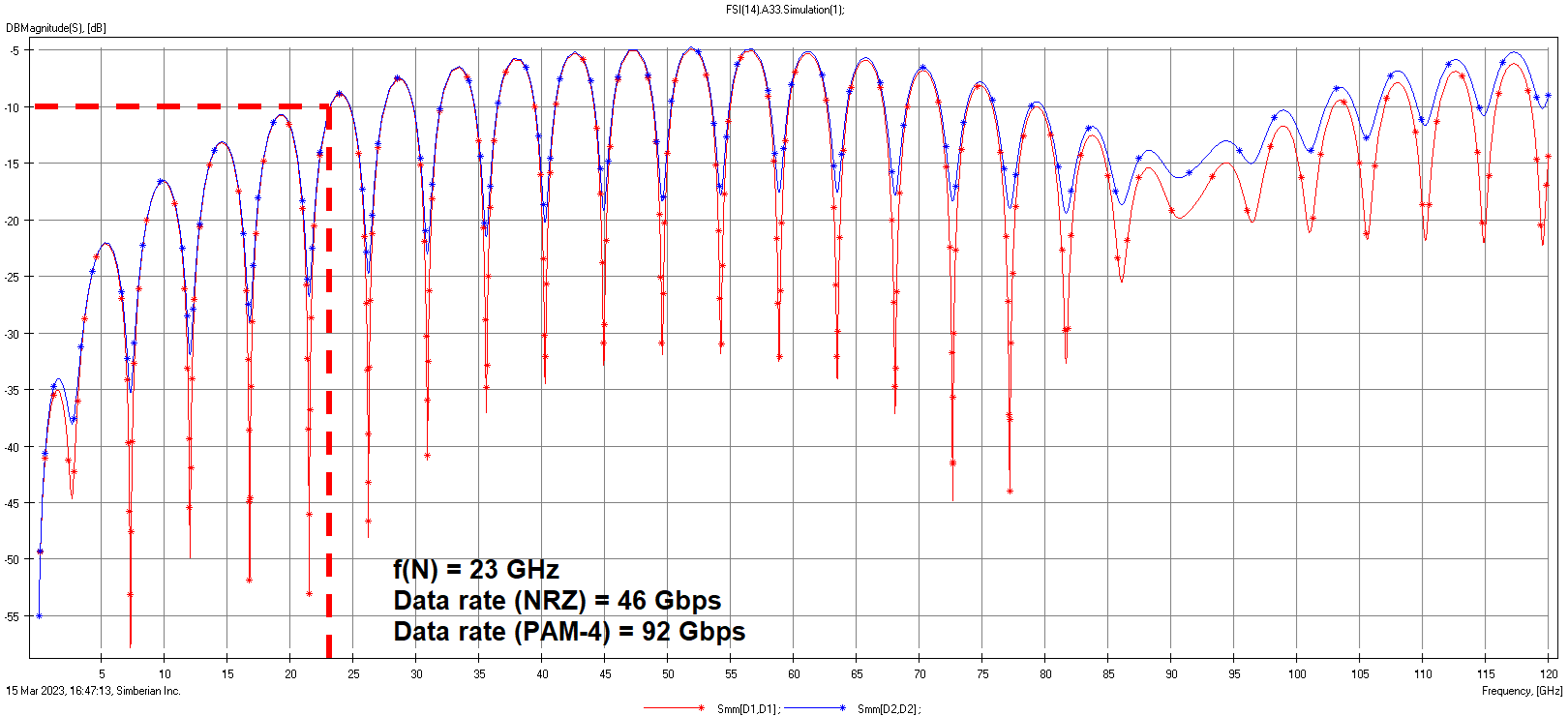Once you have an idea of the signal bandwidth, you can determine how the signal will respond to dispersion in the system and nonlinearities (e.g., in amplifying components). The need to design transmission line transfer functions or unique RF cavities to compensate for dispersion and losses seen by digital signals is a complex problem that requires numerical or analytical optimization methods to solve. Design tools should start including user-defined digital signal bandwidth in their simulation tools so that interconnects can be designed without field solvers.

At NWES, we’re experts at modeling the digital signal bandwidth and signal behavior in high speed PCB designs and sensitive analog designs. We know how to create a high quality, fully manufacturable PCB layout for your system. We're here to help electronics companies design modern PCBs and create cutting-edge technology. We've also partnered directly with EDA companies and advanced PCB manufacturers, and we'll make sure your next layout is fully manufacturable at scale. Contact NWES for a consultation.

* indicates required

## Our Clients and Partners